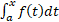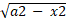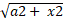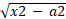﻿

Calculus, 10ed, An Indian Adaptation: One and Several Variables

ISBN: 9789390421961

1156 pages

INR 899

Description

Calculus: One and Several Variables is a classic book known for its elegant writing style, precision, and perfect balance of theory and applications. The book explores the concepts, properties, and applications of the differential and integral calculus. Starting with limits and continuity, the book focuses on derivatives and indefinite integrals of various algebraic and transcendental functions.

Chapter 1: Limits and Continuity

1.1 The Limit Process (An Intuitive Introduction)

1.2 Definition of Limit

1.3 Some Limit Theorems

1.4 Continuity

1.5 The Pinching Theorem; Trigonometric Limits

1.6 Two Basic Theorems

Chapter 2: The Derivative; The Process of Differentiation

2.1 The Derivative

2.2 Some Differentiation Formulas

2.3 The d/dx Notation; Derivatives of Higher Order

2.4 The Derivative as a Rate of Change

2.5 The Chain Rule

2.6 Differentiating the Trigonometric Functions

2.7 Implicit Differentiation; Rational Powers

Chapter 3: The Mean-Value Theorem; Applications of the First and Second Derivatives

3.1 The Mean-Value Theorem

3.2 Increasing and Decreasing Functions

3.3 Local Extreme Values

3.4 Endpoint Extreme Values; Absolute Extreme Values

3.5 Some Max-Min Problems

3.6 Concavity and Points of Inflection

3.7 Vertical and Horizontal Asymptotes; Vertical Tangents and Cusps

3.8 Some Curve Sketching

3.9 Velocity and Acceleration; Speed

3.10 Related Rates of Change Per Unit Time

3.11 Differentials

3.12 Newton-Raphson Approximations

Chapter 4: Integration

4.1 An Area Problem; A Speed-Distance Problem

4.2 The Definite Integral of a Continuous Function

4.3 The Function f(x) =4.4 The Fundamental Theorem of Integral Calculus

4.5 Some Area Problems

4.6 Indefinite Integrals

4.7 Working Back from the Chain Rule; The u-Substitution

4.8 Additional Properties of the Definite Integral

4.9 Mean-Value Theorems for Integrals; Average Value of a Function

Chapter 5: Some Applications of the Integral

5.1 More on Area

5.2 Volume by Parallel Cross Sections; Disks and Washers

5.3 Volume by the Shell Method

5.4 The Centroid of a Region; Pappus’s Theorem on Volumes

5.5 The Notion of Work

5.6 Fluid Force

Chapter 6: The Transcendental Functions

6.1 One-to-One Functions; Inverses

6.2 The Logarithm Function, Part I

6.3 The Logarithm Function, Part II

6.4 The Exponential Function

6.5 Arbitrary Powers; Other Bases

6.6 Exponential Growth and Decay

6.7 The Inverse Trigonometric Functions

6.8 The Hyperbolic Sine and Cosine

6.9 The Other Hyperbolic Functions

Chapter 7: Techniques of Integration

7.1 Integral Tables and Review

7.2 Integration by Parts

7.3 Powers and Products of Trigonometric Functions

7.4 Integrals Featuring,,7.5 Rational Functions; Partial Fractions

7.6 Some Rationalizing Substitutions

7.7 Numerical Integration

Chapter 8: Some Differential Equations

8.1 First-Order Linear Equations

8.2 Integral Curves; Separable Equations

8.3 The Equation y¢¢+ ay¢ + by = 0

Chapter 9: The Conic Sections; Polar Coordinates; Parametric Equations

9.1 Geometry of Parabola, Ellipse, Hyperbola

9.2 Polar Coordinates

9.3 Sketching Curves in Polar Coordinates

9.4 Area in Polar Coordinates

9.5 Curves Given Parametrically

9.6 Tangents to Curves Given Parametrically

9.7 Arc Length and Speed

9.8 The Area of a Surface of Revolution; The Centroid of a Curve; Pappus’s Theorem on Surface Area

Chapter 10: Sequences; Indeterminate Forms; Improper Integrals

10.1 The Least Upper Bound Axiom

10.2 Sequences of Real Numbers

10.3 Limit of a Sequence

10.4 Some Important Limits

10.5 The Indeterminate Form (0/0)

10.6 The Indeterminate Form (¥/¥); Other Indeterminate Forms

10.7 Improper Integrals

Chapter 11: Infinite Series

11.1 Sigma Notation

11.2 Infinite Series

11.3 The Integral Test; Basic Comparison, Limit Comparison

11.4 The Root Test; The Ratio Test

11.5 Absolute Convergence and Conditional Convergence; Alternating Series

11.6 Taylor Polynomials in x; Taylor Series in x

11.7 Taylor Polynomials and Taylor Series in x a

11.8 Power Series

11.9 Differentiation and Integration of Power Series

Chapter 12: Vectors in Three-dimensional Space

12.1 Rectangular Space Coordinates

12.2 Vectors in Three-Dimensional Space

12.3 The Dot Product

12.4 The Cross Product

12.5 Lines

12.6 Planes

12.7 Higher Dimensions

Chapter 13: Vector Calculus

13.1 Limit, Continuity, Vector Derivative

13.2 The Rules of Differentiation

13.3 Curves

13.4 Arc Length

13.5 Curvilinear Motion; Curvature

13.6 Vector Calculus in Mechanics

13.7 Planetary Motion

Chapter 14: Functions of Several Variables

14.1 Elementary Examples

14.2 A Brief Catalogue of the Quadric Surfaces; Projections

14.3 Graphs; Level Curves and Level Surfaces

14.4 Partial Derivatives

14.5 Open and Closed Sets

14.6 Limits and Continuity; Equality of Mixed Partials

Chapter 15: Gradients; Extreme Values; Differentials

15.3 The Mean-Value Theorem; the Chain Rule

15.4 The Gradient as a Normal; Tangent Lines and Tangent Planes

15.5 Local Extreme Values

15.6 Absolute Extreme Values

15.7 Maxima and Minima with Side Conditions

15.8 Differentials

15.9 Reconstructing a Function from its Gradient

Chapter 16: Double and Triple Integrals

16.1 Multiple-Sigma Notation

16.2 Double Integrals

16.3 The Evaluation of Double Integrals by Repeated Integrals

16.4 The Double Integral as the Limit of Riemann Sums; Polar Coordinates

16.5 Further Applications of the Double Integral

16.6 Triple Integrals

16.7 Reduction to Repeated Integrals

16.8 Cylindrical Coordinates

16.9 The Triple Integral as the Limit of Riemann Sums; Spherical Coordinates

16.10 Jacobians; Changing Variables in Multiple Integration

Chapter 17: Line Integrals and Surface Integrals

17.1 Line Integrals

17.2 The Fundamental Theorem for Line Integrals

17.3 Work-Energy Formula; Conservation of Mechanical Energy

17.4 Another Notation for Line Integrals; Line Integrals with Respect to Arc Length

17.5 Green’s Theorem

17.6 Parametrized Surfaces; Surface Area

17.7 Surface Integrals

17.8 The Vector Differential Operator

17.9 The Divergence Theorem

17.10 Stokes’ Theorem

18.1 Bernoulli Equations; Homogeneous Equations

18.2 Exact Differential Equations; Integrating Factors

18.3 Numerical Methods

18.4 The Equation y¢¢+ ay¢ + by = ϕ(x)

18.5 Mechanical Vibrations

Appendix C Tables of Integrals

Appendix C Answers to Odd Numbered Exercises

Index

• Name:
• Designation:
• Name of Institute:
• Email:
• * Request from personal id will not be entertained
• Moblie:
• ISBN / Title:
• ISBN:    * Please specify ISBN / Title Name clearly